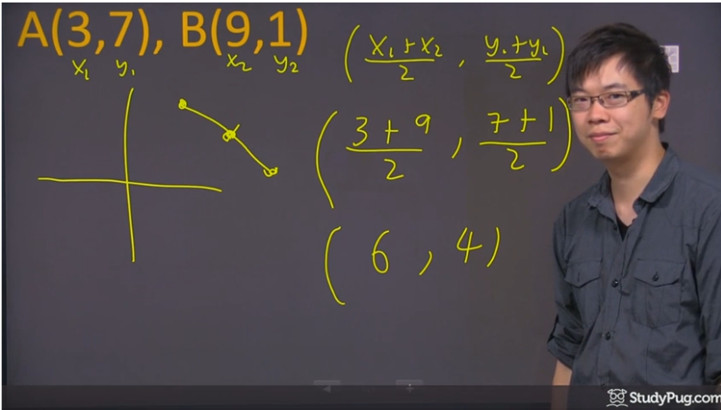# Midpoint formula: $M = ( \frac{x_1+x_2}2 ,\frac{y_1+y_2}2)$

## What is the midpoint formula?

At times, you may need to find the midpoint between two points. This usually comes into play when a question asks you to divide up a line into two equal halves, or in word problems when it asks you to find the midpoint.

If you think about it, the idea of finding the number that lies between a set of numbers is quite easy. What do you usually do? You'll take the average of them. First add them together, then divide it by two. The midpoint formula is similar and instead of taking the average of just a number, you'll have to take the average of the x- and y- values from two points separately.

The midpoint of the x-value is halfway between the two points' x-values. The midpoint of the y-value is halfway between the two points' y-values. This makes a lot of sense, right?

Of course, this means that you'll need to know the coordinates of the two points in question before you can find its midpoint. But once you know the endpoints of a line segment, you can easily find the midpoint.

This is all summed up nicely with the midpoint formula, which is as follows:

$M=(\frac{x_1+x_2}{2}, \frac{y_1+y_2}{2})$

## How to find midpoint

In order to see the midpoint formula in use, let's look at an example.

Question:

Determine the midpoint of the line segment with the given endpoints.

$A(3, 7), B(9, 1)$

Solution:

We can use the formula for midpoint to determine the midpoint: $M=(\frac{x_1+x_2}{2}, \frac{y_1+y_2}{2})$

First, plug the x and y value into the formula:

$M=(\frac{3+9}{2}, \frac{7+1}{2})$

Then, we can calculate the midpoint:

$(6, 4)$steps of calculating the midpoint

Let's take a closer look at the steps from the above example. Firstly, we are given the coordinates of the two points for which we must find the midpoint of a segment. This is all you'll need in order to make use of the midpoint formula.

First, we'll take out the x-coordinates first so that we can work on finding the midpoint's x-coordinate. We have 3 and 9 taken from point A and B respectively. Simply add the two together then divide it by 2 to get the average, a.k.a the midpoint for the x-value.

Then, do the same thing with the y-coordinates. We have 7 and 1 from, again, point A and B respectively. Adding that together gives us 8, and dividing it by two gives us 4.

We have determined that the midpoint between two points lies exactly at the coordinates (6,4) through the midpoint rule using the midpoint equation. If you plotted the line segment out onto a graph, and then dropped a point down onto (6,4), you'll indeed see that this is the point that separates the segment into two equal portions.

Another word for a point that cuts a line into two equal segments is called a bisector. Some questions may ask you to find the bisector of a line, which is basically asking you for a midpoint. You may also come across questions asking if a certain coordinate is a bisector, and you'll have to determine with the midpoint formula whether you get the midpoints that was stated. If not, then it isn't a bisector.

Check if you got the right answers by referencing this midpoint calculator if you get stuck.

### Midpoint formula: $M = ( \frac{x_1+x_2}2 ,\frac{y_1+y_2}2)$

When given the end points of a line segment, you can find out its midpoint by using the midpoint formula. As the name might have already suggested, midpoint is basically the halfway between two end points. All you need to do is dividing the sum of x-values and the sum of y-values by 2. This formula also comes in very handy when you are asked to find the point of intersection or equation of a perpendicular bisector of a given line segment.

#### Lessons

• Introduction
a)
What is the Midpoint Formula? How to use it?

• 1.
Determine the midpoint of the line segment with the given endpoints.
a)
$A(3,7), B(9,1)$

b)
$A(x+3,y-2), B(x-2,y+9)$

• 2.
Determine the missing x.
a)
$A(3,7), B(9,x)$; Midpoint $(6,4)$

b)
$A(x,2), B(3x,18)$; Midpoint $(-8,10)$